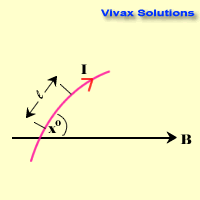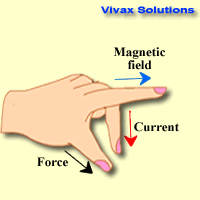### Motor Effect - loudspeaker

When an electric current flows through a conductor which is placed in the middle of a magnetic field, it is subjected to a force. This phenomenon is known as motor effect. Electric motors work on this principle that attracted this particular name for it.F = BIL sin x

F = force; B = magnetic flux density; I = current; l = length

Fleming's Left-hand Rule:Hold the thumb and the first two fingers of the left hand at right angles. Represent the magnetic field by index finger, the current by the middle finger and the thumb gives the direction of motion.

The value of the force depends on four factors:

1. Magnetic flux density - strength of magnetic field
2. Current
3. Length of the conductor
4. Angle between the conductor and the magnetic field

If the conductor is parallel to the magnetic field, there is no force acting on it -
x = 0 => sin x = 0; F = 0.

If the conductor is at right angles to the magnetic field, the force is maximum -
x = 90 => sin 90 = 1; F is maximum.

Loudspeakers show motor effect:

1. A coil surrounds a cone that is capable of moving to and fro in a limited space
2. The coil is in a magnetic field
3. when a current goes through the coil, coil along with the cone, move horizontally due to the force created by motor effect. The vibration of the cone, produces the sound

Since both 'B' and 'L' are constant, how does a loudspeaker produce different sounds?

It is all down to the current; it is a varying alternating current - AC - whose magnitude is dependent on the characteristics of the sound, that produces the current in the first place. Now we know, how a loudspeaker can produce all the different sounds.

The following animation shows just that:1. Draw magnetic field lines around two bar magnets, when north poles face each other. Where would you expect a neutral point?
2. Define magnetic flux density. Two magnetic field lines can never intersect. Explain this.
3. Two conductors carry currents upwards when placed vertically, parallel to each other. When the direction of the currents through them is the same, they tend to attract each other. Explain.
4. The length of a conductor is 6 cm and it carries a current of 4 mA. When it is placed in a magnetic field of 0.3 T, the force on the conductor varies with the angle between the conductor and the magnetic field. Sketch a graph to show how the force varies with the angle. Hence determine when the force is going to be maximum. Calculate the maximum force as well.
5. There is a horizontal wire that carries a current of 5 A. Below that lies another conductor, parallel to the above, that carries a current of 4 A. The distance between the wires is 3 cm. If the second wire can stay in balance on its own, calculate its mass per unit length. g = 9.8 ms-2.
6. Show that a moving charge (q), when travelled across a flux density (B) at velocity (v), is subjected to a force of Bqv. Show further that the charge takes the route of a circle.
7. There are circular-shaped magnets in a motor around a radial coil. Discuss the significance of this arrangement.
8. A direct current motor has a commutator to come into contact with an external power supply. Discuss the importance of this device.The best book for both teachers and students to learn physics - exactly like in the good old days:concepts are clearly explained in detail;no meaningless cartoons to devour space;the author rendered a great service in his unique approach for generations of students, with this being the fourth edition.

### GCSE Physics - Flash Cards

Amazon Best SellerPhysics flash cards have become an Amazon Best Seller; they are intuitive and summarizes the topic contents really well in beautiful layouts. They are vital for your forthcoming exams.

### A Level Physics - Flash CardsIt's not easy to find flash cards for A Level physics. These cards fill the void with lots of cards, covering the major topics that you need to know. The layout is beautiful and inspiring.